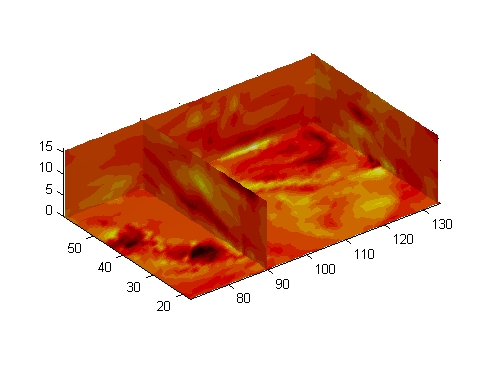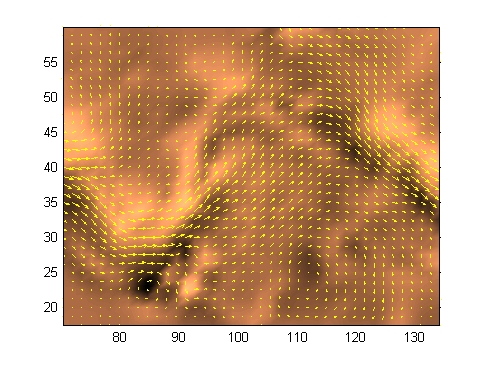MATLAB Function Referencecurl

Computes the curl and angular velocity of a vector field

Syntax

• ```[curlx,curly,curlz,cav] = curl(X,Y,Z,U,V,W)
[curlx,curly,curlz,cav] = curl(U,V,W)
[curlz,cav]= curl(X,Y,U,V)
[curlz,cav]= curl(U,V)
[curlx,curly,curlz] = curl(...), [curlx,curly] = curl(...)
cav = curl(...)
```

Description

```[curlx,curly,curlz,cav] = curl(X,Y,Z,U,V,W) ``` computes the curl and angular velocity perpendicular to the flow (in radians per time unit) of a 3-D vector field `U`, `V`, `W`. The arrays `X`, `Y`, `Z` define the coordinates for `U`, `V`, `W` and must be monotonic and 3-D plaid (as if produced by `meshgrid`).

```[curlx,curly,curlz,cav] = curl(U,V,W) ``` assumes `X`, `Y`, and `Z` are determined by the expression

• ``````[X Y Z] = meshgrid(1:n,1:m,1:p)
``````

where `[m,n,p] = size(U)`.

```[curlz,cav]= curl(X,Y,U,V) ``` computes the curl `z`-component and the angular velocity perpendicular to `z` (in radians per time unit) of a 2-D vector field `U`, `V`. The arrays `X`, `Y` define the coordinates for `U`, `V` and must be monotonic and 2-D plaid (as if produced by `meshgrid`).

```[curlz,cav]= curl(U,V) ``` assumes `X` and `Y` are determined by the expression

• ``````[X Y] = meshgrid(1:n,1:m)
``````

where `[m,n] = size(U)`.

```[curlx,curly,curlz] = curl(...), curlx,curly] = curl(...) ``` returns only the curl.

``` ``` ```cav = curl(...) ``` returns only the curl angular velocity.

Examples

This example uses colored slice planes to display the curl angular velocity at specified locations in the vector field.

• ```load wind
cav = curl(x,y,z,u,v,w);
slice(x,y,z,cav,[90 134],,);
shading interp
daspect([1 1 1]); axis tight
colormap hot(16)
camlight```

This example views the curl angular velocity in one plane of the volume and plots the velocity vectors (`quiver`) in the same plane.

• ```load wind
k = 4;
x = x(:,:,k); y = y(:,:,k); u = u(:,:,k); v = v(:,:,k);
cav = curl(x,y,u,v);
pcolor(x,y,cav); shading interp
hold on;
quiver(x,y,u,v,'y')
hold off
colormap copper```

See Also

`streamribbon`, `divergence`

Volume Visualization for related functions

Displaying Curl with Stream Ribbons for another examplecumtrapz customverctrl© 1994-2005 The MathWorks, Inc.